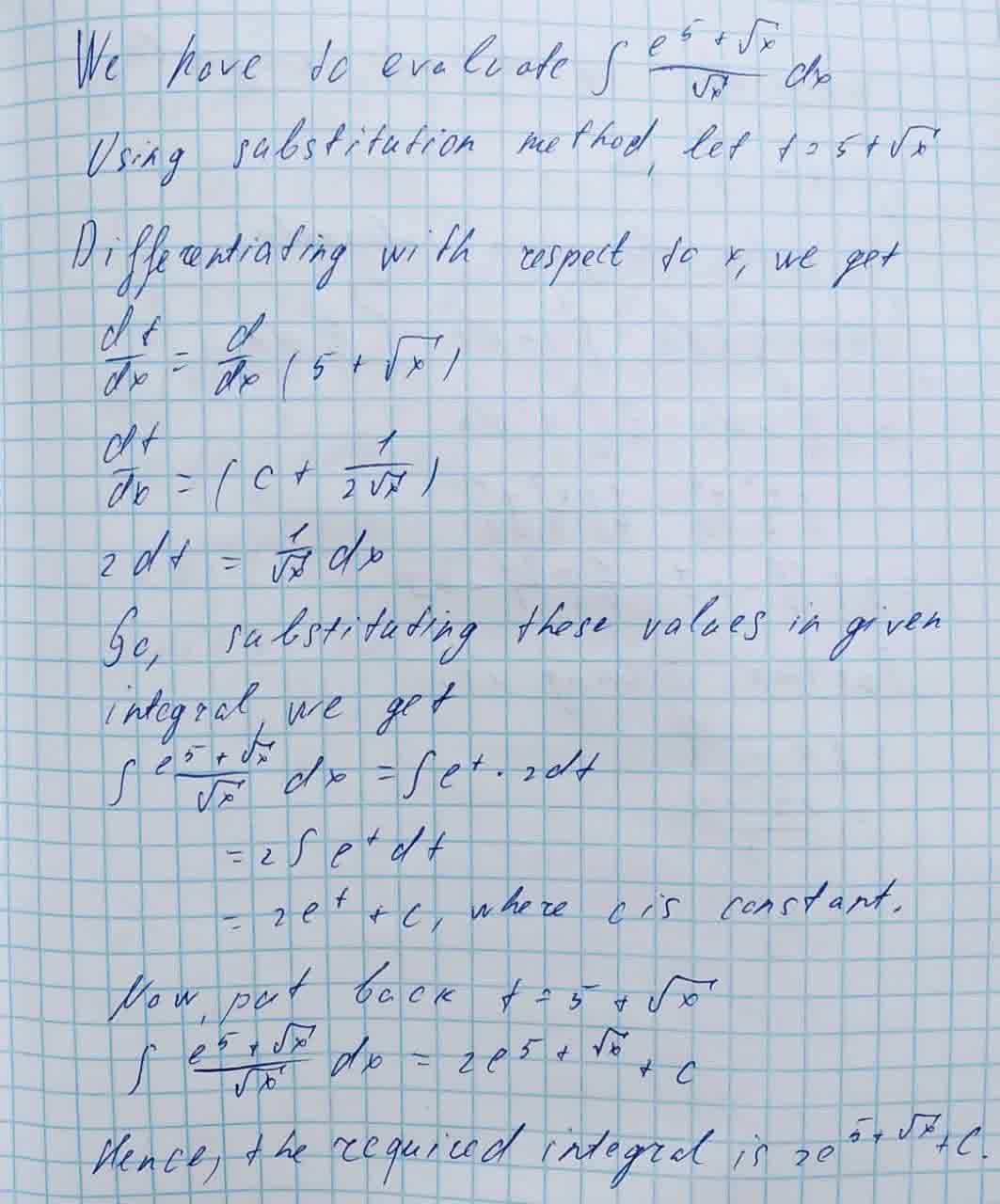Question# Evaluate the following integral \int \frac{e^{5+\sqrt{x}}}{\sqrt{x}}dx

Applications of integrals
ANSWEREDEvaluate the following integrals. Include absolute values only when needed.
$$\displaystyle\int{\frac{{{e}^{{{5}+\sqrt{{{x}}}}}}}{{\sqrt{{{x}}}}}}{\left.{d}{x}\right.}$$